Students can access the CBSE Sample Papers for Class 12 Chemistry with Solutions and marking scheme Term 2 Set 6 will help students in understanding the difficulty level of the exam.

## CBSE Sample Papers for Class 12 Chemistry Standard Term 2 Set 6 with Solutions

Max. Marks: 35
Time: 2 Hours

General Instructions:

• There are 12 questions in this question paper with internal choice.
• Section A – Q. No. 1 to 3 are very short answer questions carrying 2 marks each.
• Section B – Q. No. 4 to 11 are short answer questions carrying 3 marks each.
• Section C- Q. No. 12 is case-based question carrying 5 marks.
• All questions are compulsory.
• Use of log tables and calculators is not allowed.

Section – A

Question 1.
Arrange the following in the order of their property indicated (Any two):decreasing values of Ka.
(b) Ethanol (I), acetic acid (II), Phenol (III), Benzoic acid (IV) decreasing values of acidic strength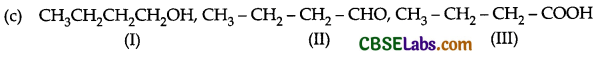increasing values of boiling point.(b) Ethanol (I)< Phenol (III)< acetic acid (II)< Benzoic acid (IV)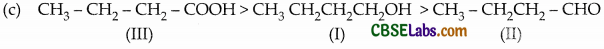Question 2.
What pressure of H2 would be required to make the e.m.f. of the hydrogen electrode zero in pure water at 25°C?
The cell reaction is:
2H+(aq) + 2e ⇌ H2(g)
According to Nemst equation, E° = E° – $$\frac{0.059}{2} \log \frac{p\left(\mathrm{H}_{2}\right)}{\left[10^{-7}\right]^{2}}$$
Since, E =0, [H+] = 10-7 M for pure water
0=0- $$\frac{0.059}{2} \log \frac{p\left(\mathrm{H}_{2}\right)}{[\mathrm{H}]^{2}}$$
∴ 0 = $$\frac{0.059}{2} \log p\left(\mathrm{H}_{2}\right)+\frac{0.059}{2} \log 10^{-14}$$
∴ log p(H2) = log 10-14
∴ p(H2) = 10 -14atm.

Enter the percent yield and theoretical yield in the below online actual yield calculator and then click calculate button to find the answer.

Question 3.
(a) The boiling points of aldehydes and ketones are lower than that of the corresponding alcohols and acids? Explain why.
(b) Oxidation of toluene to benzaldehyde with CrO3 is carried out in pressure of acetic anhydride. Why?
(a) The boiling points of aldehydes and ketones are lower than that of corresponding alcohols and acids due to absence of intermolecular hydrogen bonding in aldehydes and ketones.

(b) Oxidation of toluene to benzaldehyde is carried out with CrO3 in presence of acetic anhydride as it traps aldehyde as gem diacetate and further oxidation does not takes place to give carboxylic acid.Section – B

Question 4.
Account for the following:
(a) Tertiary amines do not undergo acylation reaction.
(b) Silver chloride dissolves in methylamine solution.
(c) Amines are more basic than comparable alcohols.
OR
Convert the following:
(a) Aniline to chlorobenzene
(b) Nitrobenzene to phenol
(c) Aniline to benzoic acid
(a) Due to the lack of acidic hydrogen, tertiary amines do not undergo acylation reaction.
(b) Methylamine can act as a base or a ligand, with silver chloride it acts as a ligand. A silver methylamine complex is formed:
AgCl + 2CH3NH2 [Ag(CH3NH2)2]+ Cl Soluble complex
(c) Lone pair of ‘N’ is easily available for donation compared to oxygen as ‘N’ is less electronegative than ‘O’. Therefore amines are more basic than corresponding alcohols.Question 5.
(a) Give an example of an organometallic compound having sandwich structure,
(b) Write IUPAC name of the following compounds—
(i) K3[Fe(C2O4)3]
(ii) [Co(NH3)6]Cl3.
OR
(a) Cu(OH)2 is soluble in NH4OH but not is NaOH solution. Why?
(b) Draw the structure of Zeise’s salt.
(c) Name the central metal atom present in hemoglobin and chlorophyll.
(a) Ferrocene
(b)
(i) Potassium trio xalatoferrate (III)
(ii) Hexaamine cobalt (III) chloride
OR
(a) Cu(OH)2 is soluble is NH4OH due to the formation of soluble complex [Cu(NH3)2](OH)2.
But no such complex is formed by the reaction of Cu(OH)2 with NaOH.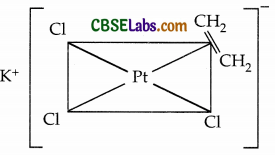(c) Iron (Fe) and Magnesium (Mg).

Question 6.
Account for the following:
(a) Europium(II) is more stable than Cerium(II)
(b) Transition elements from interstitial compounds.
(c) Separation of Zr and Hf from a mixture is difficult.
(a) Electronic configuration Eu2+ is [Xe] 4f7 5d0 while Ce2+ is [Xe]
4f25d0. Hence, Eu(II) has stable configuration as d-orbital is half filled whereas Ce2+ configuration has no such extra stability.

(b) In transition metals small size atoms like carbon, boron, nitrogen etc., occupy the interstices or holes present in the metal lattice. e.g., TiC, VH0.6, Fe3H. These compounds are more malleable, have a high melting point and are chemically inert.

(c) Separation of Zr and Hf from a mixture is difficult due to their similar atomic radii they have common physical properties due to which their separation is difficult.Question 7.
Two molecules of organic compound ‘A’ on treatment with a strong base gives two compounds ‘B’ and ‘C’. compound ‘B’ on dehydration with Cu gives A’ while acidification of ‘C’ yields carboxylic acid ‘D’ having molecular formula of CH2O2. Identify the A, B, C, D.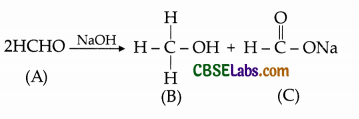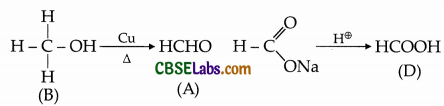Question 8.
Depict the galvanic cell in which the given reaction
Zn(s)+ 2Ag+(aq) → Zn2+(aq) + 2Ag(s) takes place. Further show:
(a) Which electrode is negatively charged?
(b) The carriers of the current in the cell.
(c) Individual reaction at each electrode.
The galvanic cell in which the given reaction takes place is1 depicted as:
Zn(s) | Zn2+(aq) || Ag+(aq) |Ag(s)
(a) Zn electrode (anode) is negative charged.
(b) Ions are carriers of current in the cell and in the external circuit, current will flow from silver to zinc.
Ions are Zn2+ and Ag+.
(c) The reaction takmg place at the anode is given by’
Zn(s) → Zn2+(aq) + 2e
The reaction taking place at the cathode is given by,
Ag+(aq) + e → Ag(s).

Question 9.
What happens when:
(a) Amines react with carboxylic acid.
(b) Aniline reacts with bromine water.
(b) Aniline reacts with cone. HNOs and cone. H2SO4.
OR
(a) Write IUPAC name of (CH3)2 CH – NH2
(b) Complete the following:(a) Amines react with carboxylic acid to form salt.(b) Aniline reacts with bromine water to form 2, 4,. 6-tribromoaniline(c) Aniline reacts with conc. HNO3 and Conc. H2SO4 to form o, m, and p-nitro aniline in different
proportions.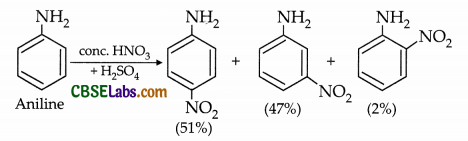OR
(a) Propan-2-amine.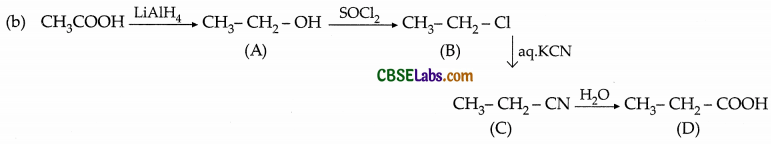Question 10.
Explain what happens when an opposing external voltage is applied on a galvanic cell when it is(a) smaller than (b) equal to (c) greater than cell potential.
(a) Cell performs normally. Electrons flow from anode to cathode and conventional current in the opposite way.
(b) No current flow, The external voltage balances cell potential, hence equilibrium is reached.
(c) The cell now acts as an electrolytic cell, zinc acts as cathode and copper acts as anode. Flow of charge is from copper to zinc and conventional current flows from zinc to copper electrode.Question 11.
(a) Why is KMnO4 solution used to clean surgical instruments in hospitals?
(b) Out of cobalt and zinc salts, which is attracted in a magnetic field. Explain with reasons.
(c) Name a transition element which does not exhibit variable oxidation states.
OR
(a) The melting and boiling points of Zn, Cd, and Hg are low. Why?
(b) Write chemical equation for the disproportionation of Mn (IV) in acidic solution.
(c) Why do Zr and Hf exhibit similar properties?
(a) KMnO4 solution is used to clean surgical instruments because of the germicidal action of KMnO4.
(b) In cobalt salts, Co has d7 electrons in the outer shell and hence it has three unpaired electrons.
Therefore it will be attracted in a magnetic field. But in case of Zr, it has got d10 configuration, and therefore, will not be attracted by magnetic field.

(c) Scandium (Z = 21) does not exhibit variable oxidation states.
OR
(a) The metallic bonds present in Zn, Cd and Hg are weak as the electrons in d-subshell are paired for them. Hence, they have low melting and boiling points.
(b) 2MnO42- +4H+ → 2MnO4 + MnO2 + 2H2O.
(c) Hf and Zn have almost similar size due to lanthanoid contraction. Hence, their properties are similar.

Section – C

Question 12.
All chemical reactions proceed through one or more transition-state intermediates whose content of free energy is greater than that of either the reactants or the products. For the simple reaction
R (reactants) ⇌ P (products),
we can write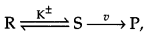, where S is the reaction intermediate with the highest free energy; K± is the equilibrium constant for the reaction R ⇌ S, the conversion of the reactant to the high-energy intermediate S; and v is the rate constant for conversion of S into the product P.

The energetic relation between the initial reactants and the products of a reaction can usually be depicted as shown in Figure given below. The free energy of activation ΔG± is equal to the difference in free energy between the transition-state intermediate S and the reactant R.

Because ΔG± generally has a very large positive value, only a small fraction of the reactant molecules will at any one time have acquired this free energy, and the overall rate of the reaction will be limited by the rate of formation of S.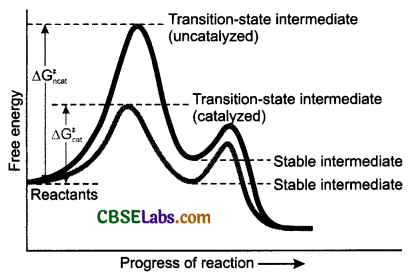(a) What is the main difference between a photosensitizer and a catalyst?
(b) Give reason, why the rate of a reaction generally increases with rise in temperature.
(c) A reaction is found to be zero order, will its molecularity be zero?
(d) A reaction is second order with respect to a reactant. How will the rate of reaction be affected if the concentration of this reactant is:
(i) doubled and
(ii) reduced to half.
OR
(d) Why order of a reaction cannot be determined by looking at the balanced chemical reaction?